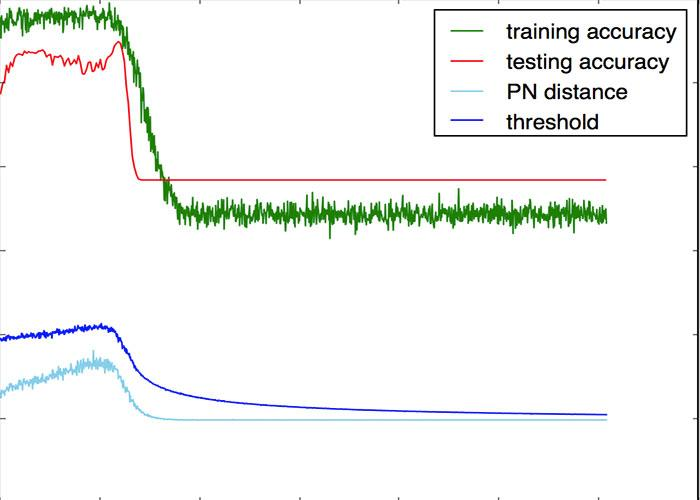## python如何画折线图

545次阅读python画折线图利用的是matplotlib.pyplot.plot的工具来绘制折线图，这里先给出一个段代码和结果图：

```# -*- coding: UTF-8 -*-
import numpy as np
import matplotlib as mpl
import matplotlib.pyplot as plt

#这里导入你自己的数据
#......
#......
#x_axix，train_pn_dis这些都是长度相同的list()

#开始画图
sub_axix = filter(lambda x:x%200 == 0, x_axix)
plt.title('Result Analysis')
plt.plot(x_axix, train_acys, color='green', label='training accuracy')
plt.plot(sub_axix, test_acys, color='red', label='testing accuracy')
plt.plot(x_axix, train_pn_dis,  color='skyblue', label='PN distance')
plt.plot(x_axix, thresholds, color='blue', label='threshold')
plt.legend() # 显示图例

plt.xlabel('iteration times')
plt.ylabel('rate')
plt.show()
#python 一个折线图绘制多个曲线```color：曲线颜色，blue，green，red等等

label：图例，这个参数内容就自定义啦，注意如果写这个参数一定要加上plt.legend()，之后再plt.show()才有有用！

linestyle：曲线风格，’–’，’-.’，’:’等等

linewidth：曲线宽度，自定义就可以

marker：标记点样式，’o’,’x’，也就是说这些符号会标示出曲线上具体的“点”，这样一来就易于观察曲线上那些地方是支撑点

markersize：标记点的大小，自定义就可以。